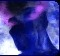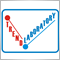# Squelch Control Indicator463

Hi,

I have the EasyLanguage code to implement the Squelch Control as a Paintbar and I need someone to write an indicator for MT4. This indicator was writen by John Ehlers. I think it's very useful.

Inputs: Price((H+L)/2),

Squelch(20);

Vars: InPhase(0),

Phase(0),

DeltaPhase(0),

count(0),

InstPeriod(0),

Period(0);

If CurrentBar > 5 then begin

Value1 = Price - Price;

Value2 =Value1;

Value3 =.75*(Value1 - Value1) + .25*(Value1 - Value1);

InPhase = .33*Value2 + .67*InPhase;

{Use ArcTangent to compute the current phase}

{Resolve the ArcTangent ambiguity}

If InPhase 0 then Phase = 180 - Phase;

If InPhase < 0 and Quadrature < 0 then Phase = 180 + Phase;

If InPhase > 0 and Quadrature < 0 then Phase = 360 - Phase;

{Compute a differential phase, resolve phase wraparound, and limit delta phase errors}

DeltaPhase = Phase - Phase;

If Phase 270 then DeltaPhase = 360 + Phase - Phase;

If DeltaPhase < 1 then DeltaPhase = 1;

If DeltaPhase > 60 then Deltaphase = 60;

{Sum DeltaPhases to reach 360 degrees. The sum is the instantaneous period.}

InstPeriod = 0;

Value4 = 0;

For count = 0 to 40 begin

Value4 = Value4 + DeltaPhase[count];

If Value4 > 360 and InstPeriod = 0 then begin

InstPeriod = count;

end;

end;

{Resolve Instantaneous Period errors and smooth}

If InstPeriod = 0 then InstPeriod = InstPeriod;

Period = .25*InstPeriod + .75*Period;

If Period < Squelch then begin

Plot1(High,"High");

Plot2(Low,"Low");

end;

end;

Files:
squelch.gif 27 kb307

So this indicator paints the bars as in your attached screen? It looks nice as long as it is not painting past bars. I would like to try it out too but unfortunately I am not a programmer :[463

Yes, it paints the bars as in attached screen but i haven't seen it "in action" so I don't know if it paints the past. I hope it doesn't.7832

Hi,

I rewrote this indicator for MQL4, but I think, that in it there is a bug.

I should check it on TS.

Igor

Files:
sci_v1.mq4 5 kb463

Hi Igor,

Thank you for the indicator.

It seems though, there is something wrong with it. It suppose to paint blue all the bars of an uptrend and red for the rest(downtrend & ranging periods). I can see lots of downtrends in blue (instead of red). Maybe there was an error in the formula I posted.

If I can find that formula elsewhere, I will post the corrections.